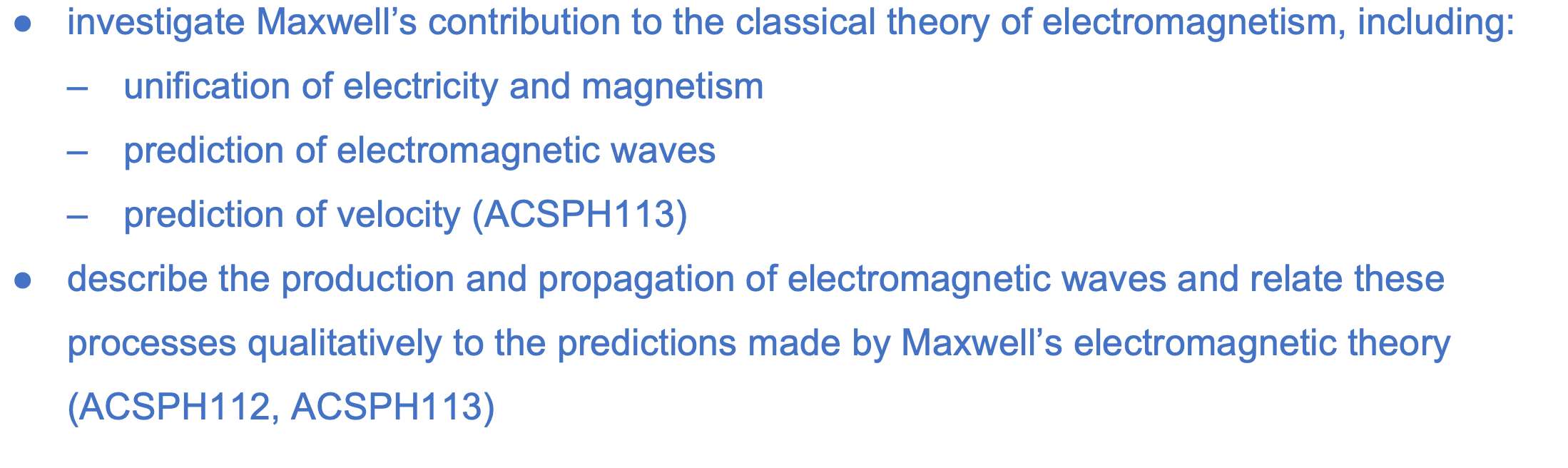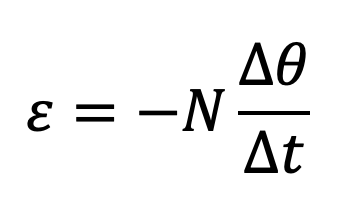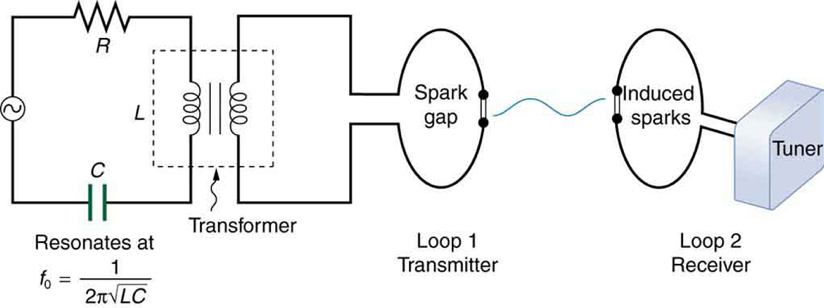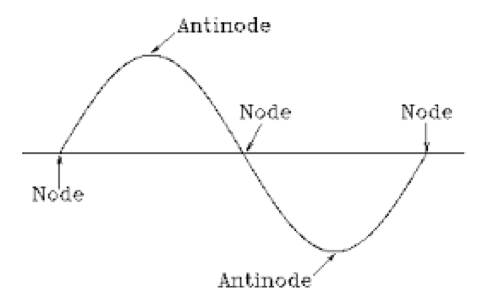# M7-S1: Maxwell's Contribution to Electromagnetism• In the 1830s, Michael Faraday proposed that changing magnetic fields produced electric fields

In Module 6: Electromagnetism, we learnt about Faraday’s Law of induction whereby when a conductor experiences change in magnetic flux (changing magnetic field), a current is induced. The induction of current resembles an electric field as electrons are directed in a certain direction that is parallel to the orientation of the electric field.• In 1861, Scottish James Maxwell extended and corrected Faraday’s proposal by postulating that changing electric fields produced magnetic fields and in fact the two phenomena should be perceived as a single entity.
In his paper ‘A Dynamic Theory of Electromagnetic Field’ (1865), he utilised previously derived mathematic equations (from his 1861 paper) to obtain a new equation that contains properties of both electric and magnetic fields. This new equation also possesses properties of a wave. As such, he postulated the idea of unifying electric and magnetic fields into one entity for simpler understanding.

• This means oscillating electric fields would produce magnetic fields. Oscillating magnetic fields would produce electric fields.
• A moving electric charge would thus produce a magnetic field due to the presence of its intrinsic electric field.

• Maxwell’s Classical Theory of Electromagnetic Waves
The new equation obtained by combining previously derived equations was characteristic of a wave. Thus, Maxwell proposed that when an oscillating electric field is present, an oscillating magnetic field would be produced perpendicular to the electric field; and both these fields would propagate in space in the same direction as a wave. This idea was Maxwell’s prediction of electromagnetic waves.Electromagnetic waves are electric and magnetic fields that propagate through space as waves while oscillating perpendicularly to one another.

In particular, Maxwell’s classical theory of electromagnetic waves refined the way scientists perceived light as it was later confirmed that light is in fact an electromagnetic wave.

• Maxwell’s classical model of light (Rayleigh and Jeans) proposes that the energy contained within light or any other electromagnetic wave is continuous and is inversely proportional to its wavelength.

• In his 1864 paper ‘Electromagnetic Theory of Light’, Maxwell used experimental findings and differential equations to calculate the speed of electromagnetic waves.
At the time, the concept of electromagnetic waves and even radio waves were absent. Maxwell utilised experimental results involving electricity and magnetism as well as his differential equations to calculate the speed of this proposed ‘wave’ (later shown to be electromagnetic wave). His equations did not contain any variables concerning light.

He determined the speed to be 310,740,000 ms-1 which is similar to the speed of light determined in the 19th century by Leon Foucault. Maxwell did not think this was a coincidence as he commented:

"We can scarcely avoid the conclusion that light consists in the transverse undulations of the same medium which is the cause of electric and magnetic phenomena."

Therefore, he predicted there was a non-coincidental link between speed of electromagnetic waves and light. In his comment, he alluded to the possibility that light may be associated with the same ‘medium’ in which electric and magnetic fields oscillate.

• Maxwell’s contribution to electromagnetism allowed for different and more accurate methods to be used to calculate the speed of light.

Rosa and Dorsay in 1907 indirectly determined the speed of light through using the electric and magnetic permeability of air.

• This positive finding verified the existence of electromagnetic waves and confirmed that light in fact does have electromagnetic properties.
• The value attained from this experiment had to be later corrected because speed of electromagnetic waves was deduced to be independent of the medium they propagate in.

• The German physicist Heinrich Hertz was the first to generate and detect certain types of electromagnetic waves in the laboratory. He performed a series of experiments that not only confirmed the existence of electromagnetic waves, but also verified that they travel at the speed of light.Figure 2: Hertz's experimental set-up.

Hertz used an AC circuit that resonates at a known frequency and connected it to a loop of wire as shown in Figure 2. High voltages induced across the gap in the loop produced sparks that were visible evidence of the current in the circuit and that helped generate electromagnetic waves.

• Frequency of alternating current = frequency of oscillating electric field = frequency of oscillating magnetic field = frequency of electromagnetic wave produced.

Across the laboratory, Hertz had another loop attached to another circuit, which could be tuned (as the dial on a radio) to the same resonant frequency as the first and could, thus, be made to receive electromagnetic waves. This loop also had a gap across which sparks were generated, giving solid evidence that electromagnetic waves had been received.

Hertz also studied the reflection, refraction, and interference patterns of the electromagnetic waves he generated, verifying their wave character.From determining the distances between nodes, he was able to determine wavelength from the interference patterns, and knowing their frequency, he could calculate the propagation speed using the equation:

Hertz was thus able to prove that electromagnetic waves travel at the speed of light. The SI unit for frequency, the hertz (1 Hz = 1 cycle/sec), is named in his honor.
• The contribution of Maxwell to electromagnetism inspired Heinrich Hertz in 1888 to discover the Photoelectric Effect.
Hertz created self-induction devices from metal plates and coils which would generate sparks between spark balls (shown below). These sparks are essentially accelerated electrons (charged particles) migrating between the spark balls through air.Hertz conducted this experiment initially to verify the existence of electromagnetic waves and to his observation, sparks were formed between the spark balls of a nearby circular coil. This coil was not connected to any potential difference. Hertz proposed that the sparks (electrons) generated electromagnetic waves that stimulated the induction of sparks at the circular coil.

However, he was unable to fully explain why only sparks of certain frequency can induce sparks at the receiving coil, regardless of their intensity.

﻿Next Section: ﻿Speed of Light